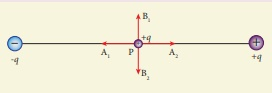# TN 12TH PHYSICS - Online Test

Q1. In which of the following , NH3 is not used?
Explaination / Solution:
No Explaination.

Q2. Sc( Z=21) is a transition element but Zinc (z=30) is not because
Explaination / Solution:
No Explaination.

Q3. The sum of primary valance and secondary valance of the metal M in the complex [ M ( en)2 ( Ox)] Cl is L
Explaination / Solution:

In the complex [M (en)2 (Ox)] Cl For the central metal ion M3+

The primary valance is = +3

The secondary valance = 6

sum of primary valance and secondary valance = 3+6 = 9

Q4. Graphite and diamond are
Explaination / Solution:
No Explaination.

Q5. For a first order reaction A → B the rate constant is x min1 . If the initial concentration of A is 0.01M , the concentration of A after one hour is given by the expression.
Explaination / Solution:In this case

k = x min−1 and [A0] = 0.01M = 1 × 10−2M

t = 1 hour = 60 min

[A]= 1 × 10−2 ( e−60x)

Q6. The speed of light in an isotropic medium depends on,
Explaination / Solution:

c = vλ, v is independent of medium

Q7. A plane glass is placed over a various coloured letters (violet, green, yellow, red) The letter which appears to be raised more is,
Explaination / Solution:

The refractive index (n) of the glass is different for different colours. For violet n is high. ∴ Violet travel with low speed, so deviation is high.

Q8. Two identical point charges of magnitude –q are fixed as shown in the figure below. A third charge +q is placed midway between the two charges at the point P. Suppose this charge +q is displaced a small distance from the point P in the directions indicated by the arrows, in which direction(s) will +q be stable with respect to the displacement?Explaination / Solution:

Hint: Since V=0 along equatorial line
Q9. The wavelength λe of an electron and λp of a photon of same energy E are related by
Explaination / Solution:

de Broglie wavelength for an electron

λe= h / √(2mE) ;

λe 1/√E ;

λe2 1/E  ------------- (1)

For photon

λp= hc / E ;

λp 1/E  ------------- (2)

From equation (1) and (2), we have So,

λp λe2

Q10. The following graph shows current versus voltage values of some unknown conductor. What is the resistance of this conductor?Explaination / Solution: